# Preface

This section presents some properties of the most remarkable and useful in numerical computations Chebyshev polynomials of first kind $$T_n (x)$$ and second kind $$U_n (x) .$$ Both Chebyshev polynomials are eigenfunctions of the corresponding singular Sturm--Liouville problems. Other two Chebyshev polynomials of the third kind and the fourth kind are not so popular in applications.

Introduction to Linear Algebra with Mathematica

# Chebyshev expansions

Interpolation with Chebyshev nodes (where the Chebyshev polynomial is zero, that is, cos \left( \frac{2i-1}{2n} \.\pi \right)) is easily computed in Matlab >> f = @(x) 1./(1+25*x.^2); >> n = 11; >> xe = linspace(-1,1,n); >> xc = cos((2*(1:n)-1)*pi/2/n); >> t = -1:.01:1; >> plot(t,f(t),'b',t,neville(xe,f(xe),t),'r',xe,f(xe),’o’) >> plot(t,f(t),'b',t,neville(xc,f(xc),t),'r',xc,f(xc),’o’)

The Chebyshev polynomials are named after a Russian mathematician Pafnuty Lvovich Chebyshev (1821--1894), who invented them in 1859 while solving a practical problem for converting horizontal movement of piston in stream engine into rotational one. Of course, contemporary rail road engines have nothing in common with old steam engines first used in 19 century. His name can be alternatively transliterated as Chebychev, Chebysheff, Chebychov, Chebyshov; or Tchebychev, Tchebycheff (French transcriptions); or Tschebyschev, Tschebyschef, Tschebyscheff, Tschebyschow (German transcriptions). He also used two Russian spellings: Чебышёв and Чебышо́в.

# Chebyshev Polynomials

There are known four kinds of Chebyshev polynomials that could be defined through generating functions:

\begin{align*} \frac{1-xt}{1-2xt + t^2} &= \sum_{n\ge 0} T_n (x)\, t^n , \\ \frac{1}{1-2xt + t^2} &= \sum_{n\ge 0} U_n (x)\, t^n , \\ \frac{1-t}{1-2xt + t^2} &= \sum_{n\ge 0} V_n (x)\, t^n , \\ \frac{1+t}{1-2xt + t^2} &= \sum_{n\ge 0} W_n (x)\, t^n . \end{align*}
However, the first two, Tn and Un, are most popular because they are eigenfunctions of the following Sturm--Liouville problems, respectively:
\begin{align*} \left( 1 - x^2 \right) y'' - x\, y' + \lambda\,y &=0 , \qquad \lambda = n^2 , \\ \left( 1 - x^2 \right) y'' - 3x\, y' + \lambda\,y &=0 , \qquad \lambda = n(n+2) . \end{align*}
These polynomials could be defined "explicitly" either by the hypergeometric series (which becomes a finite sum)
\begin{align*} T_n (x) &= _2F_1 \left( -n, n, \frac{1}{2}; \frac{1-x}{2} \right) = \frac{n}{2} \sum_{k=0}^{\left\lfloor \frac{n}{2} \right\rfloor} \frac{(-1)^k}{n-k} \binom{n-k}{k} \left( 2x \right)^{n-2k} , \qquad n\ge 1, \\ U_n (x) &= \left( n+1 \right) _2F_1 \left( -n, n+2, \frac{3}{2}; \frac{1-x}{2} \right) = \sum_{k=0}^{\left\lfloor \frac{n}{2} \right\rfloor} (-1)^k \binom{n-k}{k} \left( 2x \right)^{n-2k} , \qquad n\ge 0, \\ V_n (x) &= _2F_1 \left( -n, n+1, \frac{1}{2}; \frac{1-x}{2} \right) = \sum_{k=0}^{n} (-1)^k \binom{2n-k}{k} 2^{n-k} \left( x-1 \right)^{n-k} , \qquad n\ge 0, \\ W_n (x) &= \left( 2n+1 \right) _2F_1 \left( -n, n+1, \frac{3}{2}; \frac{1-x}{2} \right) = \left( 2n+1 \right) \sum_{k=0}^{n} \frac{2^{n-k}}{2n-2k+1} \binom{2n-k}{k} \left( x-1 \right)^{n-k} , \qquad n\ge 0; \end{align*}
or by Rodrigues' formulas:
\begin{align*} T_n (x) &= \frac{(-1)^n 2^n n!}{(2n)!}\,\frac{{\text d}^n}{{\text d} x^n} \left( 1- x^2 \right)^{n-1/2} , \\ U_n (x) &= \frac{(-1)^n 2^n (n+1)!}{(2n+1)!}\left( 1 - x^2 \right)^{-1} \frac{{\text d}^n}{{\text d} x^n} \left( 1- x^2 \right)^{n+1/2} , \\ \left( 1-x \right)^{-1/2} \left( 1+x \right)^{1/2} V_n (x) &= \frac{(-1)^n 2^n n!}{(2n)!}\,\frac{{\text d}^n}{{\text d} x^n} \left( 1- x \right)^{n-1/2} \left( 1+ x \right)^{n+1/2}, \\ \left( 1-x \right)^{1/2} \left( 1+x \right)^{-1/2} W_n (x) &= \frac{(-1)^n 2^n n!}{(2n)!}\,\frac{{\text d}^n}{{\text d} x^n} \left( 1- x \right)^{n+1/2} \left( 1+ x \right)^{n-1/2} . \end{align*}
The first two Chebyshev polynomials admit explicit expressions:
\begin{align*} T_n (x) &= \begin{cases} \cos \left( n\,\mbox{arccos}\,x \right) , & \ |x| \le 1, \\ \frac{1}{2} \left( x + \sqrt{x^2 -1} \right)^n + \frac{1}{2} \left( x - \sqrt{x^2 -1} \right)^n , & \ |x| \ge 1, \end{cases} \\ U_n &= \frac{1}{2\sqrt{x^2 -1}} \left[ \left( x + \sqrt{x^2 -1} \right)^{n+1} - \left( x - \sqrt{x^2 -1} \right)^{n+1} \right] . \end{align*}

The Chebyshev polynomials satisfy orthogonalities with respect to various weight functions as in the following:

\begin{align*} \int_{-1}^1 \left( 1 - x^2 \right)^{-1/2} T_n (x) T_m (x) \,{\text d}x &= \frac{\pi}{{\cal E}_n} \, \delta_{n,m} , \\ \int_{-1}^1 \left( 1 - x^2 \right)^{1/2} U_n (x) U_m (x) \,{\text d}x &= \frac{\pi}{2} \, \delta_{n,m} , \\ \int_{-1}^1 \left( \frac{1+x}{1-x} \right)^{1/2} V_n (x) V_m (x) \,{\text d}x &= \pi \, \delta_{n,m} , \\ \int_{-1}^1 \left( \frac{1-x}{1+x} \right)^{1/2} W_n (x) W_m (x) \,{\text d}x &= \pi \, \delta_{n,m} , \end{align*}
where
$\delta_{n,m} = \begin{cases} 0, & \ \mbox{if } n \ne m , \\ 1 , & \ \mbox{if } n = m ; \end{cases} \qquad\quad {\cal E}_n = \begin{cases} 1 , & \ \mbox{if } n =0, \\ 2, & \ \mbox{if } n \ge 1. \end{cases}$

There are two kinds of Chebyshev expansions for a function on the finite interval [-1, 1] depending which kind of Chebyshev function is used. The Chebyshev polynomials of first kind $$T_n (x)$$ are solutions of the differential equation

$\left( 1- x^2 \right) y'' -x\,y' + n^2 y =0 ,$
and they are orthogonal on the interval [-1, 1] with weight function $$\rho (x) = \left( 1- x^2 \right)^{-1/2} :$$
$\int_{-1}^1 \frac{T_m (x)\, T_n (x)}{\sqrt{1-x^2}} \,{\text d} x = \begin{cases} \pi , & \quad \mbox{if n=m=0}, \\ \pi /2 , & \quad \mbox{if n=m\ne 0}, \\ 0 , & \quad \mbox{if n\ne m}. \end{cases}$
The Chebyshev polynomials of the second kind $$U_n (x)$$ are solutions of the differential equation
$\left( 1- x^2 \right) y'' -3x\,y' + n(n+2)\, y =0 ,$
and they are orthogonal on the interval [-1, 1] with weight function $$\rho (x) = \left( 1- x^2 \right)^{1/2} :$$
$\int_{-1}^1 U_m (x)\, U_n (x)\, \sqrt{1-x^2} \,{\text d} x = \begin{cases} \pi /2 , & \quad \mbox{if n=m}, \\ 0 , & \quad \mbox{if n\ne m}. \end{cases}$
Both Chebyshev differential equations can be reduced to constant coefficient equations by substitutions either $$x= \cos t$$ or $$x= \cosh t$$ depending whether |x| is less than 1 or greater than 1. Chebyshev polynomials satisfy the identities:
$T_n \left( \cos \theta \right) = \cos n\theta \qquad \mbox{and} \qquad U_n \left( \cos \theta \right) \sin \theta = \sin (n+1)\theta , \quad \theta \in [0, \pi ], \quad n=0,1,2,\ldots .$
Chebyshev polynomials lead to different explicit expressions such as:
\begin{align*} T_n (x) &= \frac{1}{2} \left[ \left( x + \sqrt{x^2 -1} \right)^n + \left( x - \sqrt{x^2 -1} \right)^n \right] \qquad \mbox{for} \quad |x| \ge 1, \\ &= \begin{cases} \cos \left( n\,\mbox{arccos} x \right) , & \quad \mbox{if $|x| \le 1$}, \\ \cosh \left( n\,\mbox{arccosh} x \right) , & \quad \mbox{if $x\ge 1$}, \\ (-1)^n \cosh \left( n\,\mbox{arccosh} (-x) \right) , & \quad \mbox{if $x\le -1$}; \end{cases} \\ &= x^n \sum_{k=0}^{\lfloor n/2 \rfloor} \,\binom{n}{2k} \left( 1- x^{-2} \right)^k . \end{align*}
\begin{align*} U_n (x) &= \frac{1}{2\,\sqrt{x^2 -1}} \left[ \left( x + \sqrt{x^2 -1} \right)^{n+1} - \left( x - \sqrt{x^2 -1} \right)^{n+1} \right] = \frac{\sinh \left( (n+1)\,\mbox{arccosh} \,x \right)}{\sinh \left( \mbox{arccosh} \,x \right)} \qquad \mbox{for} \quad |x| \ge 1, \\ &= \frac{\sin \left( (n+1)\,\mbox{arccos} \,x \right)}{\sin \left( \mbox{arccos} \,x \right)} = \frac{\sin \left( (n+1)\,\mbox{arccos} \,x \right)}{\sqrt{1-x^2}} \quad \mbox{for } |x| \le 1; \\ &= x^n \sum_{k=0}^{\lfloor n/2 \rfloor} \,\binom{n+1}{2k+1} \left( 1- x^{-2} \right)^k . \end{align*}
Shifted Chebyshev polynomials are also of interest when the range of the independent variable is [0,1] instead of [-1, 1]. The shifted Chebyshev polynomials are defined as
$T_{n}^{\ast} (x) = T_n (2x-1) , \qquad U_{n}^{\ast} (x) = U_n (2x-1) , \qquad n=0,1,2,\ldots .$
There are known also third- and fourth-kind Chebyshev polynomials:
$V_{n} (x) = \frac{\cos \left( \frac{2n+1}{2}\,\theta \right)}{\cos \left( \frac{\theta}{2} \right)} , \qquad W_n (x) = \frac{\sin \left( \frac{2n+1}{2}\,\theta \right)}{\sin \left( \frac{\theta}{2} \right)} , \qquad x= \cos\theta , \qquad n=0,1,2,\ldots .$

Most of the properties of Chebyshev polynomials follow from their generating functions:
$\frac{1- tx}{1-2xt + t^2} = \sum_{n\ge 0} T_n (x)\, t^n , \qquad \frac{1}{1-2xt + t^2} = \sum_{n\ge 0} U_n (x)\, t^n .$
Recurrence relations:
\begin{align*} T_{n+1} (x) &= 2x\,T_n (x) - T_{n-1} (x) , \quad n=2,3,\ldots ; \quad T_0 (x) =1 , \quad T_1 (x) =x; \\ U_{n+1} (x) &= 2x\,U_n (x) - U_{n-1} (x) , \quad n=2,3,\ldots ; \quad T_0 (x) =1 , \quad T_1 (x) =2x . \end{align*}
Chebyshev polynomials of even order have even symmetry and contain only even powers of x. Chebyshev polynomials of odd order have odd symmetry and contain only odd powers of x:
$T_n (-x) = (-1)^n T_n (x) , \qquad U_n (-x) = (-1)^n U_n (x) .$
Some numerical values:
$T_n (1) = 1 , \qquad T_n (-1) = (-1)^n , \qquad T_n (0) = U_n (0) = \begin{cases} (-1)^k , & \quad \mbox{if n=2k is even}, \\ 0 , & \quad \mbox{if n=2k+1 is odd}; \end{cases} \qquad U_n (1) = n+1, \qquad U_n (-1) = (-1)^{\lfloor n/2 \rfloor} \left( n+1 \right) .$

Derivatives:

$\frac{{\text d}\,T_n (x)}{{\text d} x} = n\, U_{n-1} (x) , \qquad \frac{{\text d}\,U_n (x)}{{\text d} x} = \frac{1}{x^2 -1} \left[ (n+1)\, T_n (x) -x\,U_n (x) \right] .$
A Chebyshev polynomial of either kind with degree n has n different simple roots, called Chebyshev roots, in the interval [−1,1]. The roots of the Chebyshev polynomial of the first kind are sometimes called Chebyshev nodes because they are used as nodes in polynomial interpolation. The extrema of Chebyshev polynomials of first kind are located at
$x_k = \cos \left( \frac{k\pi}{n} \right) , \qquad k=0,1,2,\ldots , n ;$
while zeroes of $$T_n (t)$$ are located at
$t_k = \cos \left( \frac{2k+1}{2n}\,\pi \right) , \qquad k=0,1,2,\ldots , n-1.$

Using Mathematica, we define the Chebyshev polynomials of the first kind from its generating function:

CT[n_, x_] :=
Module[{F, z, Dn}, F = ((1 - z^2)/(1 - 2*x*z + z^2) + 1)/2;
Dn = D[F, {z, n}];
Expand[Dn/n! /. z -> 0]]

Another option using the recurrence:

CT[n_, x_] :=
CT[n, x] =
If[n == 0, 1,
If[n == 1, x,
Expand[2*x*ChebyshevT[n - 1, x] - ChebyshevT[n - 2, x]]]]

Another option to define the Chebyshev polynomials directly through explicit formula:

CT[n_, x_] := Module[{k, tmp, tab}, If[n == 0, Return];
If[n == 1, Return[x]];
tmp = (2*x)^n/2;
tab = Table[
tmp = -tmp/4/k*(n - 2*k + 2)*(n - 2*k + 1)/x^2/(n - k), {k, 1, Floor[n/2]}];
(2*x)^n/2 + Apply[Plus, tab]]

# Chebyshev Series

Chebyshev polynomials form a special class of polynomials especially suited for approximating other functions. They are widely used in many areas of numerical analysis: uniform approximation, least-squares approximation, numerical solution of ordinary and partial differential equations (the so-called spectral or pseudospectral methods), and so on. In the appropriate Sobolev space, the set of Chebyshev polynomials form an orthonormal basis, so that a function in the same space can, on −1 ≤ x ≤ 1 be expressed via the expansion
$f(x) = \frac{a_0}{2} + \sum_{k\ge 1} a_k T_k (x) = \sum_{k\ge 0} a_k U_k (x) = \sum_{k\ge 0} c_k V_k (x) = \sum_{k\ge 0} d_k W_k (x) .$
The Chebyshev polynomials form an orthogonal basis which (among other things) implies that the coefficients can be determined easily through the application of an inner product. This sum is called a Chebyshev series or a Chebyshev expansion. All of the theorems, identities, etc. that apply to Fourier series have a Chebyshev counterpart.

Arbitrary continuous function can be approximated by Chebyshev interpolation and Chebyshev series that converges pointwise. If f(x) is continuous in the interval [−1,1] apart from a finite number of step discontinuities in the interior, then its Chebyshev series expansion converges to f wherever f is continuous, and to the average of the left and right limiting values at each discontinuity. To obtain uniform convergence of the Chebyshev series, continuous function f should have bounded variation, similar to Fourier series (Dirichlet or Dini--Lipschitz condition). If a function is no more than barely continuous, then we can derive uniformly convergent approximations from its Chebyshev expansion by averaging out the partial sums, and thus forming ‘Cesàro sums’ of the Chebyshev series. For the case of solutions of linear ordinary differential equations with polynomial coefficients (a typical case for special functions), the problem of computing Chebyshev series is efficiently solved by means of Clenshaw’s method.

For the sake of uniform approximation, Chebyshev series based on the Chebyshev polynomials of the first kind are the most useful ones and have faster uniform convergence:

$f(x) = \frac{c_0}{2} + \sum_{k\ge 1} \,c_k\, T_{k} (x ) ,$
where
$c_k = \frac{2}{\pi}\,\int_{-1}^1 \,\frac{f(x)\, T_{k} (x )}{\sqrt{1-x^2}}\, {\text d} x = \frac{2}{\pi}\,\int_{0}^{\pi} \, f\left( \cos \,\theta \right) \cos \left( k\,\theta \right) {\text d} \theta , \qquad k=0,1,2,\ldots .$

We can interpolate arbitrary polynomial $$P_n (x)$$ of degree n:

$P_n (x) = \,\sum_{k=0}^{n} \,^{\prime} \, c_{k} \, T_{k} (x) = \frac{c_0}{2} + \sum_{k= 1}^n \,c_k\, T_{k} (x ) ,$
where the prime indicates that the term for k = 0 is to be halved and

$c_{k} = \frac{2}{n+1} \,\sum_{j=0}^n \,P(x_j )\, T_{k} (x_j ) , \qquad x_j = \cos \left( \frac{2j+1}{2n+2}\, \pi \right) .$
We are tempted to expand a function into the series
$f(x) = \sum_{k\ge 0} \,b_k\, U_{k} (x ) ,$
where
$b_k = \frac{2}{\pi}\,\int_{-1}^1 \,f(x)\, U_{k} (x )\,\sqrt{1-x^2}\, {\text d} x = \frac{2}{\pi}\,\int_{0}^{\pi} \, f\left( \cos \,\theta \right) \sin \left( (k+1)\,\theta \right) \sin \theta \,{\text d} \theta , \qquad k=0,1,2,\ldots .$
If function g(x) is continuous on the interval [-1,1] and vanish at end points so that $$g(x)/\sqrt{1-x^2}$$ is quadratically/absolutely integrable on the interval, then we can expand it as
$g(x) = \sqrt{1-x^2} \,\sum_{k\ge 0} \,b_k\, U_{k} (x ) ,$
where
$b_k = \frac{2}{\pi}\,\int_{-1}^1 \,g(x)\, U_{k} (x )\, {\text d} x , \qquad k=0,1,2,\ldots .$

Example: The power function xn can be expressed in terms of Chebyshev polynomials as follows:

$x^n = 2^{1-n} \,\sum_{k=0}^{\lfloor n/2 \rfloor} \,^{\prime} \, \binom{n}{k} \, T_{n-2k} (x) = T_n (x) + 2^{1-n} \,\sum_{k=1}^{\lfloor n/2 \rfloor} \, \binom{n}{k} \, T_{n-2k} (x) , \qquad n=1,2,\ldots ;$
where the prime indicates that the term for k = 0 is to be halved. Similarly,

$x^n = 2^{-n} \left[ U_n (x) + \left( n-1\right) U_{n-2} (x) + \cdots \right] , \qquad n=1,2,\ldots .$

Example: Consider a continuous function $$f(x) = 4\,x^3 -1 .$$ Since it is a polynomial of degree n = 3, we can use the corresponding formula to determine the coefficients in Chebyshev series:

$c_0 = \frac{2}{4} \,\sum_{j=0}^3 \,f\left( \cos \frac{2j+1}{8}\,\pi \right) = \frac{2}{\pi}\,\int_{0}^{\pi} \, f\left( \cos \,\theta \right) {\text d} \theta = -2,$
$c_1 = \frac{2}{4} \,\sum_{j=0}^3 \,f\left( \cos \frac{2j+1}{8}\,\pi \right) T_1 \left( \cos \frac{2j+1}{8}\,\pi \right) = \frac{2}{\pi}\,\int_{0}^{\pi} \, f\left( \cos \,\theta \right) \cos \theta \, {\text d} \theta = 3,$
$c_2 = \frac{2}{4} \,\sum_{j=0}^3 \,f\left( \cos \frac{2j+1}{8}\,\pi \right) T_2 \left( \cos \frac{2j+1}{8}\,\pi \right) = \frac{2}{\pi}\,\int_{0}^{\pi} \, f\left( \cos \,\theta \right) \cos 2\theta \, {\text d} \theta = 0,$
$c_3 = \frac{2}{4} \,\sum_{j=0}^3 \,f\left( \cos \frac{2j+1}{8}\,\pi \right) T_3 \left( \cos \frac{2j+1}{8}\,\pi \right) = \frac{2}{\pi}\,\int_{0}^{\pi} \, f\left( \cos \,\theta \right) \cos 3\theta \, {\text d} \theta = 1.$
Therefore, we get the Chebyshev expansion of the given function:
$4x^3 -1 = -1 + 3\, T_1 (x) + T_3 (x) = -1 +3x + \left( 4\,x^3 -3x \right) ,$
which is an exact equation. We do similar job with Chebyshev polynomials of the second kind:
$4\,x^3 -1 = b_0 \,U_0 + b_1 \,U_1 (x) + b_2 \, U_2 (x) + b_3 \, U_3 (x) = b_0 + b_1 \,2x + b_2 \left( 4x^2 -1 \right) + b_3 \left( 8x^3 -4x \right) ,$
where
\begin{align*} b_0 &= \frac{2}{\pi} \,\int_{-1}^1 \left( 4x^3 -1 \right) \sqrt{1-x^2} \,{\text d} x = \frac{2}{\pi} \,\int_{0}^{\pi} \left( 4\cos^3 \theta -1 \right) \sin^2 \theta \, {\text d} \theta = -1, \\ b_1 &= \frac{2}{\pi} \,\int_{-1}^1 \left( 4x^3 -1 \right) \sqrt{1-x^2} \,2x\, {\text d} x = \frac{2}{\pi} \,\int_{0}^{\pi} \left( 4\cos^3 \theta -1 \right) \sin \theta \,\sin 2\theta {\text d} \theta = 1, \\ b_2 &= \frac{2}{\pi} \,\int_{-1}^1 \left( 4x^3 -1 \right) \sqrt{1-x^2} \,2x\, {\text d} x = \frac{2}{\pi} \,\int_{0}^{\pi} \left( 4\cos^3 \theta -1 \right) \sin \theta \,\sin 3\theta {\text d} \theta = 0, \\ b_3 &= \frac{2}{\pi} \,\int_{-1}^1 \left( 4x^3 -1 \right) \sqrt{1-x^2} \,2x\, {\text d} x = \frac{2}{\pi} \,\int_{0}^{\pi} \left( 4\cos^3 \theta -1 \right) \sin \theta \,\sin 4\theta {\text d} \theta = \frac{1}{2} . \end{align*}
Therefore,
$4\,x^3 -1 = -U_0 + U_1 (x) + \frac{1}{2} \, U_3 (x) = -1 + 2x + \frac{1}{4} \left( 8x^3 -4x \right) ,$
which is the identity.    ■

Example: Consider an exponential function $$f(x) = e^x .$$ Its third order Chebyshev approximation is

$e^x \approx \frac{c_0}{2} + \sum_{k=1}^3 c_k T_k (x) .$
We determine the coefficients by integration:
\begin{align*} c_0 &= \frac{2}{\pi} \, \int_0^{\pi} \, e^{\cos \theta} \, {\text d} \theta = 2\, I_0 (1) \approx 2.53213 , \\ c_1 &= \frac{2}{\pi} \, \int_0^{\pi} \, e^{\cos \theta} \,\cos \theta \, {\text d} \theta = 2\, I_1 (1) \approx 1.13032 , \\ c_2 &= \frac{2}{\pi} \, \int_0^{\pi} \, e^{\cos \theta} \,\cos 2\theta \, {\text d} \theta = 2\, I_2 (1) \approx 0.271495 , \\ c_3 &= \frac{2}{\pi} \, \int_0^{\pi} \, e^{\cos \theta} \,\cos 2\theta \, {\text d} \theta = 2\, I_3 (1) \approx 0.0443368 , \end{align*}
where $$I_n (1)$$ is the modified Bessel function of order n evaluated at x = 1. When we plot Chebyshev approximation along with the given function, we cannot determine any difference, which means that the error is less than 1%. Indeed, its mean square error is about 0.000029615.
c3 = (2/Pi)*Integrate[Exp[Cos[theta]]*Cos[3*theta], {theta , 0, Pi}]
approx = c0/2 + c1*ChebyshevT[1, x] + c2*ChebyshevT[2, x] + c3*ChebyshevT[3, x]
Plot[{Exp[x], approx}, {x, -1, 1}, PlotStyle -> {Blue, Orange}]
NIntegrate[(Exp[x] - approx)^2, {x, -1, 1}]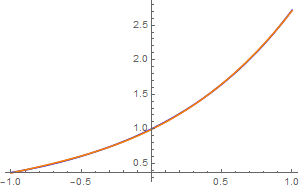ChebyshevApprox[n_Integer?Positive, f_Function, x_] :=
Module[{c, xk}, xk = Pi (Range[n] - 1/2)/n;
c[j_] = 2*Total[Cos[j*xk]*(f /@ Cos[xk])]/n;
Total[Table[c[k]*ChebyshevT[k, x], {k, 0, n - 1}]] - c/2];

f = Exp[#] &
ChebyshevApprox[3, f, x] // Simplify
Out= 1/3 (3 - E^(-(Sqrt/2)) (Sqrt - 2 x) x - 4 x^2 + E^(Sqrt/2) x (Sqrt + 2 x))
GraphicsGrid[
Partition[
Table[Plot[{f[x], ChebyshevApprox[n, f, x]}, {x, -1, 1},
Frame -> True, Axes -> False, PlotStyle -> {Blue, Red},
PlotRange -> {-2, 10},
Epilog -> Text["n = " <> ToString[n], {0.25, 5}]], {n, 9}], 3],
ImageSize -> 500]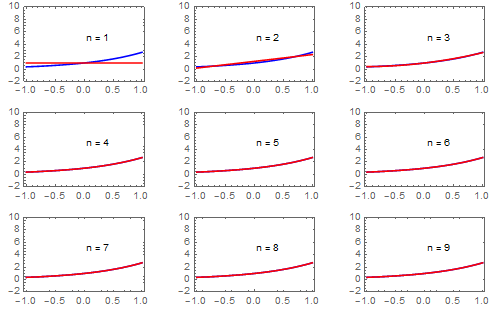So we see that all approximations with $$n \ge 3$$ terms give very good approximations. Now we use Chebyshev polynomials of the second kind:
$e^x \approx \sum_{k=0}^n b_k U_k (x) ,$
where
$b_k = \int_{-1}^1 e^x \,U_k (x)\, \sqrt{1-x^2} \, {\text d} x = 2\left( k+1 \right) I_{k+1} (1), \quad k=0,1,2,\ldots .$
Therefore, on the interval [-1,1], we have
$e^x = 2\,\sum_{k\ge 0} \left( k+1 \right) I_{k+1} (1) \, U_k (x) .$
Upon plotting finite sum approximation, we see that approximation with three terms is enough. Its mean square error is about 0.0000268334.
exp[m_, x_] := 2*Sum[(k + 1)*BesselI[k + 1, 1]*ChebyshevU[k, x], {k, 0, m}]
Plot[{Exp[x], exp[2, x]}, {x, -1.01, 1.01}, PlotStyle -> {{Thick, Blue}, {Thick, Red}},Epilog -> Text["n = " <> ToString, {-0.75, 2}]
NIntegrate[(Exp[x] - exp[3, x])^2, {x, -1, 1.0}]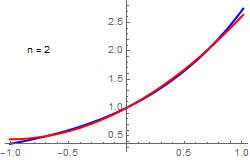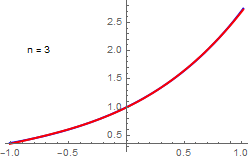Exponential approximation with N = 2 and N = 3 terms. ■

Example: Consider a problem for expanding the Heaviside function into Chebyshev's series:

$H(t) = \frac{c_0}{2} + \sum_{k\ge 1} c_k T_k (t) .$
The coefficients can be evaluated explicitly:
\begin{align*} c_0 &= \frac{2}{\pi} \, \int_0^1 \frac{{\text d}x}{\sqrt{1- x^2}} =1, \\ c_n &= \frac{2}{\pi} \, \int_0^1 \frac{T_n (x)\,{\text d}x}{\sqrt{1- x^2}} = \left\{ \begin{array}{ll} 0 , & \ \mbox{if $n=2k$ is even} \\ (-1)^k \,\frac{2}{n\pi} , & \ \mbox{if $n = 2k+1$ is odd. } \end{array} \right. \end{align*}
Therefore,
$H(t) = \frac{1}{2} + \frac{2}{\pi} \,\sum_{k\ge 0} \frac{(-1)^k}{2k+1}\, T_{2k+1} (t) .$
We plot its approximation with 11 terms:
heaviside[m_, t_] = 1/2 + (2/Pi)* Sum[(-1)^k *ChebyshevT[2*k + 1, t]/(2*k + 1) , {k, 0, m}];
Plot[{HeavisideTheta[t], heaviside[10, t]}, {t, -1.01, 1.01}, PlotStyle -> {Blue, Orange}]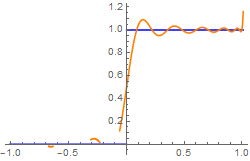We repeat calculations with Chebyshev polynomials of the second kind:
$H(t) = \frac{1}{2} + \frac{4}{\pi} \, \sum_{k\ge 0} \frac{(-1)^k\, k}{(2k+1)(2k+3)}\, U_{2k+1} (t) .$
The following graph presents 10 term approximation with Chebyshev polynomials of second kind.
chebsum[m_] := 1/2 - (4/Pi)* Sum[(-1)^k *k/(2*k - 1)/(2*k + 1) *ChebyshevU[2*k - 1, t], {k, 1, m}]
Plot[{HeavisideTheta[t], chebsum}, {t, -1.01, 1.01}, PlotStyle -> {Blue, Orange}]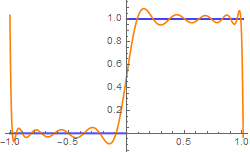We observe Gibbs phenomenon near point of discontinuity t = 0 in both expansions with respect to Chebyshev polynomials of the first and second kind, as well as bad convergence at end points.    ■

Example: Let us consider the signum function on the closed interval [-1,1], which we expand into Chebyshev series:

\begin{align*} \mbox{sign}(x) = \sum_{n\ge 1} a_n T_n (x) = \sum_{n\ge 1} b_n U_n (x) = \sum_{n\ge 0} c_n V_n (x) = \sum_{n\ge 0} d_n W_n (x) , \end{align*}
where
\begin{align*} a_n &= - \frac{2}{\pi} \int_{-1}^0 \frac{T_n (x)}{\sqrt{1-x^2}} \, {\text d}x + \frac{2}{\pi} \int_0^1 \frac{T_k (x)}{\sqrt{1-x^2}} \, {\text d}x = \frac{4}{\pi} \times \begin{cases} \frac{1}{n} , & \ \mbox{ if $n$ is odd}, \\ 0 , & \ \mbox{ if $n$ is even}; \end{cases} \\ b_n &= - \frac{2}{\pi} \int_{-1}^0 U_n (x) \,\sqrt{1-x^2} \, {\text d}x + \frac{2}{\pi} \int_0^1 U_n (x) \,\sqrt{1-x^2} \, {\text d}x = \frac{8}{\pi} \times \begin{cases} 0, & \ \mbox{if $n$ is even}, \\ \frac{(-1)^k k}{(2k-1)(2k+1)} , & \ \mbox{ if $n= 2k-1$ is odd}; \end{cases} \\ c_n &= \frac{1}{\pi} \int_0^1 \left( \frac{1+x}{1-x} \right)^{1/2} V_n (x) \, {\text d}x - \frac{1}{\pi} \int_{-1}^0 \left( \frac{1+x}{1-x} \right)^{1/2} V_n (x) \, {\text d}x = \\ d_n &= \frac{1}{\pi} \int_0^1 \left( \frac{1-x}{1+x} \right)^{1/2} W_n (x) \, {\text d}x - \frac{1}{\pi} \int_{-1}^0 \left( \frac{1+x}{1-x} \right)^{1/2} W_n (x) \, {\text d}x = \end{align*}
We plot partial sums using Mathematica:
SA[x_] = (4/Pi)*Sum[ChebyshevT[2*k - 1, x]/(2*k - 1), {k, 0, 10}] Plot[SA[x], {x, -1, 1}]
CT[n_, x_] := Piecewise[{{1, -1 < x < 0}, {0, 0}, {-1, 0 < x < 1}}];
CT[n, x] = Piecewise[{{1, -1 < x < 0}, {0, 0}, {-1, 0 < x < 1}}];
If[n == -1, 1];
Expand[2*x*ChebyshevT[n - 1, x] - ChebyshevT[n - 2, x]];
CCT0[x_] = unitstep[Mod[x, Pi, -Pi]];
ct[x_] = Piecewise[{{1, -1 <= x < 0}, {-1, 0 < x <= 1}}];
ct6ff0 = FourierSinSeries[CCT0[x], x, 6];
ctff0[x_, nmax_] := Sum[-Sin[(2 n + 1) .65 x]/(2 n + 1), {n, 0, nmax}] 1.275
stepplot[n_] := Plot[{ct[x], ChebyshevT[1, ctff0][x, n]}, {x, -1, 1}, Ticks -> {{-1, -.5, 0, .5, 1}}, PlotStyle -> {{Thick, Blue}, {Thick, Red}}, Exclusions -> None]
stepplot
stepplot
■

Example: Some other examples of Chebyshev series:

\begin{align*} \frac{\pi}{2}\left( 1 - x^2 \right)^{1/2} &= 1 - \sum_{k\ge 1} \frac{1}{2k^2 -1} \,T_{2k} (x) , \\ \mbox{sign}(x) &= \frac{4}{\pi} \,\sum_{k \ge 1} \frac{(-1)^k}{2k-1} \,T_{2k-1} (x) , \\ |x| &= \frac{2}{\pi} - \frac{4}{\pi} \,\sum_{k\ge 1} \frac{(-1)^k}{4k^2 -1} \,T_{2k} (x) \\ &= \frac{1}{2} - \sum_{k\ge 1} (-1)^k \frac{(2k-3)!!}{(2k+2)!!} \, (4k+1)\,P_{2k} (x) , \\ \mbox{arccos}(x) &= \frac{\pi}{2} - \frac{4}{\pi} \,\sum_{k\ge 1} \frac{1}{(2k-1)^2}\, T_{2k-1} (x) , \\ \mbox{arcsin}(x) &= \frac{4}{\pi} \,\sum_{k\ge 1} \frac{1}{(2k-1)^2}\, T_{2k-1} (x) . \end{align*}
1. Clenshaw, C.W., Norton, H.J.: The solution of nonlinear ordinary differential equations in chebyshev series. The Computer Journal, 1963, {\bf 6}, Issue 1, 88–92; https://doi.org/10.1093